# Introduction

In this example analysis, I will demonstrate how to run an analysis of heterogeneous treatment effects in an observational setting using the methods of Kennedy (2020).

This analysis will focus on using machine learning ensembles (through SuperLearner) to estimate nuisance functions and then provide a tibble of estimates of conditional treatment effects along with their associated standard errors.

# Load packages and setup environment

library(tidyhte)
library(ggplot2)
library(dplyr)

# Simulate data

If real data is used, simply replace this block with an appropriate readr::read_csv call or equivalent, creating a tibble. I will assume this tibble is stored as data for the remainder of this document.

Note that datatypes can be either continuous or discrete, and that there can be columns in the tibble that are not included in any resulting anayses.

set.seed(100)
n <- 500
data <- tibble(
uid = 1:n
) %>%
mutate(
x1 = rnorm(n),
x2 = factor(sample(1:4, n, prob = c(1 / 100, 39 / 100, 1 / 5, 2 / 5), replace = TRUE)),
x3 = factor(sample(1:3, n, prob = c(1 / 5, 1 / 5, 3 / 5), replace = TRUE)),
x4 = (x1 + rnorm(n)) / 2,
x5 = rnorm(n),
ps = plogis(x1 * 0.3 - as.double(x2) * 0.25 + x5 * 0.5),
a = rbinom(n, 1, ps),
y = (
a + x1 - a * (x1 - mean(x1)) + (4 * rbinom(n, 1, 0.5) - 1) * a * (x2 == 2) +
a * (x2 == 3) + 0.5 * a * (x2 == 4) +
0.25 * rnorm(n)
),
w = 0.1 + rexp(n, 1 / 0.9)
)

# Define Recipe

## Propensity score and Outcome Model

We estimate the propensity score and outcome (T-learner) plugin estimates using an ensemble of machine learning models, including a wide array of model complexities from linear models, GAMs, regularized regressions. In this example, non-linear models are not included (due to runtime), but they could easily be added by uncommenting the associated lines.

Each individual component of the model provides a list of hyperparameters, over which a full cross-product is taken and all resulting models are estimate. For instance, SL.glmnet sweeps over one hyperparameter (the mixing parameters between ridge and Lasso). A model with each of the hyper-parameter values will be estimated and incorporated into the ensemble. Note that SL.glmnet automatically tunes the regularization parameter using cv.glmnet, so this is not included as a hyperparameter.

## Quantities of interest

Quantities of Interest determine how results are reported to the user. You can think about this as determining, for instance how results should be plotted in a resulting chart.

For simplicity, this example simply provides results in one of two ways: - Discrete covariates are stratified and the conditional effect is plotted at each distinct level of the covariate. - Continuous covariates have the effect surface estimated using local-linear regression via nprobust of Calonico, Cattaneo and Farrell (2018). See, similarly, Kennedy, Ma, McHugh and Small (2017) for justification of this approach. Results are obtained for a grid of 100 quantiles across the domain of the covariate.

An additional quantity of interest provided is the variable importance of a learned joint model of conditional effects (over all covariates). The approach implemented is described in Williamson, Gilbert, Carone and Simon (2020).

hte_cfg <- basic_config() %>%
"SL.glmnet.interaction", alpha = c(0, 1)
) %>%
add_outcome_model("SL.glmnet", alpha = c(0, 1)) %>%
add_outcome_model("SL.glmnet.interaction", alpha = c(0, 1)) %>%
add_effect_model("SL.glmnet", alpha = c(0, 1)) %>%
add_effect_model("SL.glmnet.interaction", alpha = c(0, 1)) %>%
hte_cfg

# Estimate Models

To actually perform the estimation, the following will be sufficient. Note that the configuration of covariate names at the top of the document makes all of this a little more complex with all the curly-brackets and bangs.

data %>%
attach_config(hte_cfg) %>%
make_splits(uid, .num_splits = 3) %>%
produce_plugin_estimates(
y,
a,
x1, x2, x3, x4, x5,
) -> prepped_data

construct_pseudo_outcomes(prepped_data, y, a) %>%
estimate_QoI(x1, x2, x3, x4, x5) -> results
results
## # A tibble: 853 × 6
##    estimand term  value level estimate std_error
##    <chr>    <chr> <dbl> <chr>    <dbl>     <dbl>
##  1 MCATE    x1    -1.79 <NA>      4.07     0.556
##  2 MCATE    x1    -1.72 <NA>      3.95     0.507
##  3 MCATE    x1    -1.63 <NA>      3.78     0.438
##  4 MCATE    x1    -1.53 <NA>      3.62     0.382
##  5 MCATE    x1    -1.43 <NA>      3.44     0.324
##  6 MCATE    x1    -1.34 <NA>      3.29     0.280
##  7 MCATE    x1    -1.25 <NA>      3.15     0.245
##  8 MCATE    x1    -1.20 <NA>      3.07     0.227
##  9 MCATE    x1    -1.17 <NA>      3.02     0.218
## 10 MCATE    x1    -1.13 <NA>      2.96     0.206
## # ℹ 843 more rows

# ATEs

filter(results, grepl("SATE|PATE", estimand))
## # A tibble: 1 × 6
##   estimand term  value level estimate std_error
##   <chr>    <chr> <dbl> <chr>    <dbl>     <dbl>
## 1 SATE     <NA>     NA <NA>      1.79     0.121

# Plots

## Plot Ensemble Coefficients

filter(results, grepl("SL coefficient", estimand)) %>%
mutate(level = factor(level, levels = c("Control Response", "Treatment Response"))) %>%
ggplot(aes(
x = reorder(term, estimate),
y = estimate,
ymin = estimate - 1.96 * std_error,
ymax = estimate + 1.96 * std_error
)) +
geom_abline(intercept = 0, slope = 0, linetype = "dashed") +
geom_pointrange() +
expand_limits(y = 0) +
scale_x_discrete("Model name") +
scale_y_continuous("Coefficient in SuperLearner Ensemble") +
facet_wrap(~level) +
coord_flip() +
ggtitle("SuperLearner Ensemble") +
theme_minimal()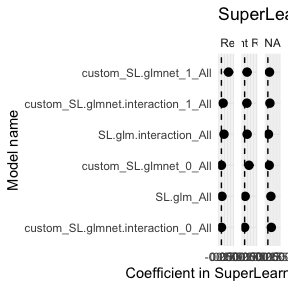## Plot risk for each submodel

filter(results, grepl("SL risk", estimand)) %>%
mutate(
level = factor(level, levels = c("Control Response", "Treatment Response", "Effect Surface"))
) %>%
ggplot() +
geom_abline(intercept = 0, slope = 0, linetype = "dashed") +
geom_pointrange(
aes(
x = reorder(term, -estimate),
y = estimate,
ymin = estimate - 1.96 * std_error,
ymax = estimate + 1.96 * std_error)
) +
expand_limits(y = 0) +
scale_x_discrete("Model name") +
scale_y_continuous("CV Risk in SuperLearner Ensemble") +
facet_wrap(~level, scales = "free_x") +
coord_flip() +
ggtitle("Submodel Risk Estimates") +
theme_minimal()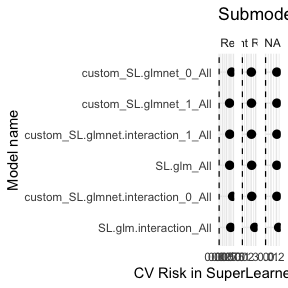## Plot Regression ROC Curves

filter(results, grepl("RROC", estimand)) %>%
mutate(
level = factor(level, levels = c("Control Response", "Treatment Response", "Effect Surface"))
) %>%
ggplot() +
geom_line(
aes(
x = value,
y = estimate
)
) +
geom_point(
aes(x = value, y = estimate),
data = filter(results, grepl("RROC", estimand)) %>% group_by(level) %>% slice_head(n = 1)
) +
expand_limits(y = 0) +
scale_x_continuous("Over-estimation") +
scale_y_continuous("Under-estimation") +
facet_wrap(~level, scales = "free_x") +
coord_flip() +
ggtitle("Regression ROC Curves") +
theme_minimal()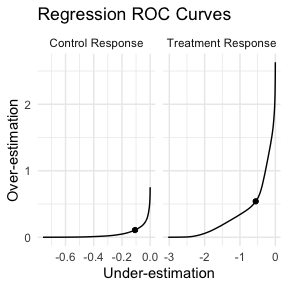## Plot VIMP

ggplot(filter(results, estimand == "VIMP")) +
geom_abline(intercept = 0, slope = 0, linetype = "dashed") +
geom_pointrange(
aes(
x = term,
y = estimate,
ymin = estimate - 1.96 * std_error,
ymax = estimate + 1.96 * std_error
)
) +
expand_limits(y = 0) +
scale_x_discrete("Covariate") +
scale_y_continuous("Reduction in R² from full model") +
coord_flip() +
ggtitle("Covariate Importance") +
theme_minimal()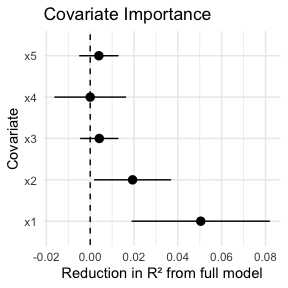## Plot Continuous Covariates’ MCATE

for (cov in c("x1", "x4", "x5")) {
ggplot(filter(results, estimand == "MCATE", term == cov)) +
geom_abline(intercept = 0, slope = 0, linetype = "dashed") +
geom_ribbon(
aes(
x = value,
ymin = estimate - 1.96 * std_error,
ymax = estimate + 1.96 * std_error
),
alpha = 0.75
) +
geom_line(
aes(x = value, y = estimate)
) +
expand_limits(y = 0) +
scale_x_continuous("Covariate level") +
scale_y_continuous("CATE") +
ggtitle(paste("Marginal effects across", cov)) +
theme_minimal() -> gp
print(gp)
}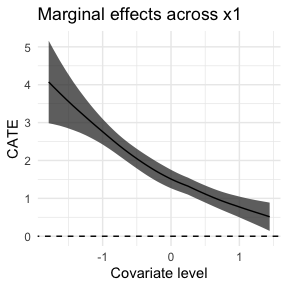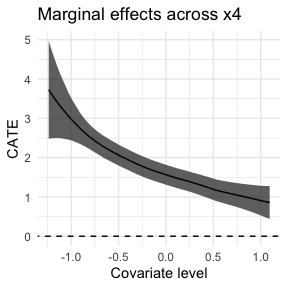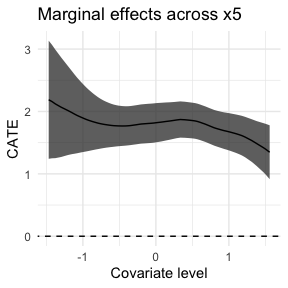## Plot Discrete Covariates’ MCATE

for (cov in c("x2", "x3")) {
ggplot(filter(results, estimand == "MCATE", term == cov)) +
geom_abline(intercept = 0, slope = 0, linetype = "dashed") +
geom_pointrange(
aes(
x = level,
y = estimate,
ymin = estimate - 1.96 * std_error,
ymax = estimate + 1.96 * std_error
)
) +
expand_limits(y = 0) +
scale_x_discrete("Covariate level") +
scale_y_continuous("CATE") +
ggtitle(paste("Marginal effects across", cov)) +
theme_minimal() -> gp
print(gp)
}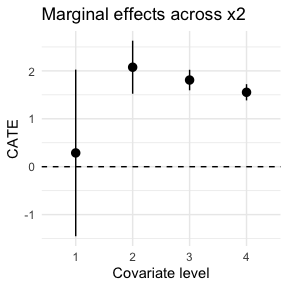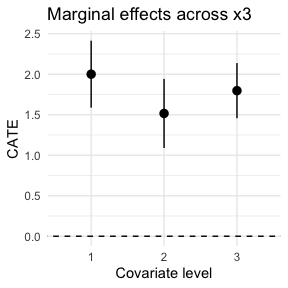# Session Info

print(sessionInfo())
## R version 4.3.1 (2023-06-16)
## Platform: aarch64-apple-darwin22.4.0 (64-bit)
## Running under: macOS Ventura 13.4.1
##
## Matrix products: default
## BLAS:   /opt/homebrew/Cellar/openblas/0.3.23/lib/libopenblasp-r0.3.23.dylib
## LAPACK: /opt/homebrew/Cellar/r/4.3.1/lib/R/lib/libRlapack.dylib;  LAPACK version 3.11.0
##
## locale:
##  C/en_US.UTF-8/en_US.UTF-8/C/en_US.UTF-8/en_US.UTF-8
##
## time zone: Europe/Vienna
## tzcode source: internal
##
## attached base packages:
##  stats     graphics  grDevices utils     datasets  methods   base
##
## other attached packages:
##  magrittr_2.0.3        palmerpenguins_0.1.1  nnls_1.4
##  SuperLearner_2.0-28.1 dplyr_1.1.2           ggplot2_3.4.2
##  tidyhte_1.0.2
##
## loaded via a namespace (and not attached):
##   vimp_2.3.1            sass_0.4.6            utf8_1.2.3
##   generics_0.1.3        quickblock_0.2.0      shape_1.4.6
##   lattice_0.21-8        distances_0.1.9       hms_1.1.3
##  digest_0.6.33         evaluate_0.21         grid_4.3.1
##  iterators_1.0.14      fastmap_1.1.1         Matrix_1.5-4.1
##  glmnet_4.1-7          foreach_1.5.2         jsonlite_1.8.7
##  progress_1.2.2        backports_1.4.1       survival_3.5-5
##  purrr_1.0.1           fansi_1.0.4           scales_1.2.1
##  codetools_0.2-19      jquerylib_0.1.4       cli_3.6.1
##  rlang_1.1.1           crayon_1.5.2          scclust_0.2.3
##  munsell_0.5.0         splines_4.3.1         withr_2.5.0
##  cachem_1.0.8          yaml_2.3.7            tools_4.3.1
##  checkmate_2.2.0       colorspace_2.1-0      boot_1.3-28.1
##  nprobust_0.4.0        vctrs_0.6.3           R6_2.5.1
##  lifecycle_1.0.3       MASS_7.3-60           pkgconfig_2.0.3
##  pillar_1.9.0          bslib_0.5.0           gtable_0.3.3
##  glue_1.6.2            data.table_1.14.8     Rcpp_1.0.11
##  highr_0.10            xfun_0.39             tibble_3.2.1
##  tidyselect_1.2.0      knitr_1.43            farver_2.1.1
##  htmltools_0.5.5       labeling_0.4.2        rmarkdown_2.23
##  prettyunits_1.1.1     WeightedROC_2020.1.31